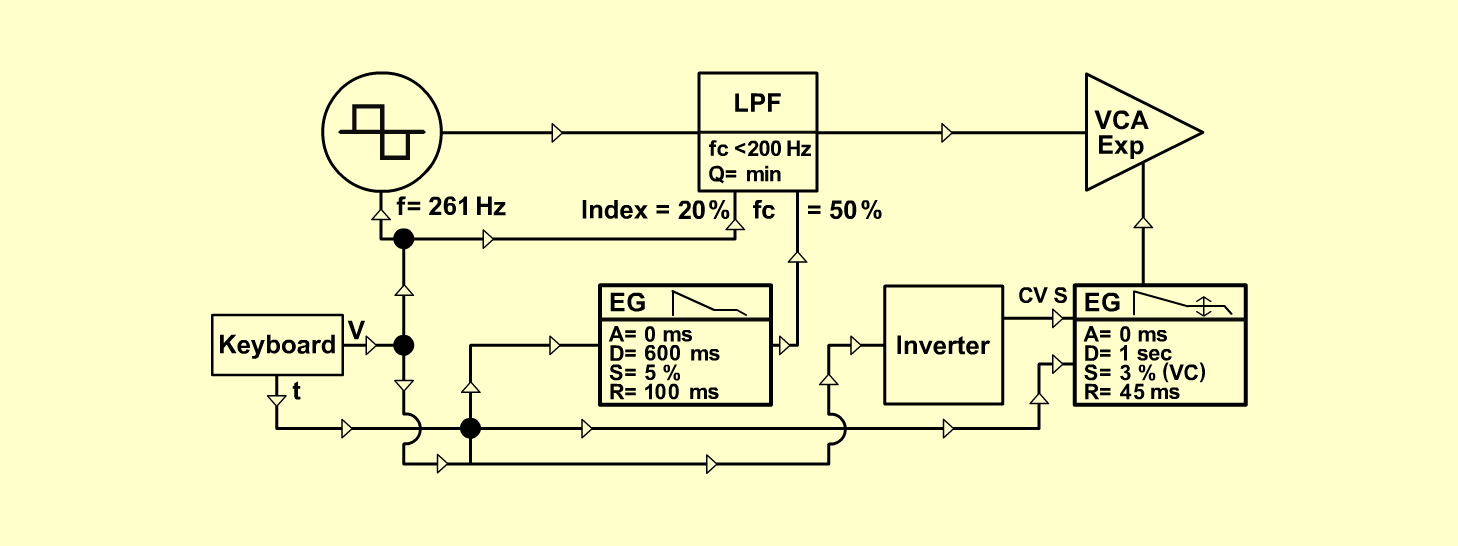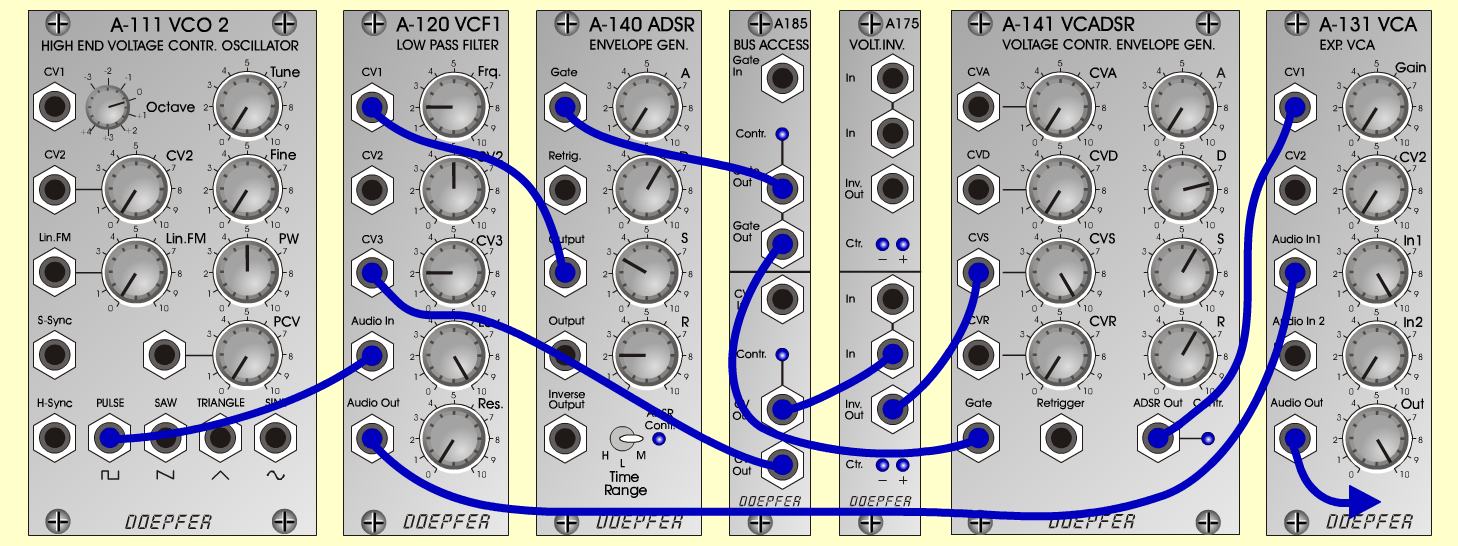HarpInstrumentsD o e p f e r A - 100 Connections: Settings: A-111 (Square)  <=>  A-120 (Audio In) A-120 (Audio Out)  <=>  A-131 (Audio In 1) A-185 (Gate Out/1)  <=>  A-140 (Gate) A-185 (Gate Out/2)  <=>  A-141 (Gate) A-185 (CV Out/1)  <=>  A-120 (CV 3) A-185 (CV Out/2)  <=>  A-175 (In) A-175 (Inv Out)  <=>  A-141 (CVS) A-140 (Output)  <=>  A-120 (CV 2) A-141 (Out)  <=>  A-131 (CV 1) A-131 (Output)  <=>  Amplifier A-111 (PW = 5) A-120 (Freq = 2, CV 2 = 5, CV 3 = 2, Lev = 10, Res = 0) A-140 (A = 0, D = 6, S = 3, R = 2, Range = M) A-141 (CV A = 0, CV D = 0, CV S = 10, CV R = 0, A = 0, D = 7.5, S = 6, R = 6) A-131 (Gain = 0, Audio In 1 = 10,  Audio Out = 10) Josef MuellerSound samples Notturno (Anonymous) Mozart (KV 299) Harp (130 Hz) Harp (262 Hz) Harp (523 Hz)